Function Repository Resource:

# TreeCounts

Create a tree of the counts of the distinct data of each subtree

Contributed by: Ian Ford (Wolfram Research)
 ResourceFunction["TreeCounts"][tree] replaces the data of each subtree of tree with an association whose keys are the distinct data of that subtree and whose values give the number of times those data appear in that subtree.

## Examples

### Basic Examples (1)

Give a tree of the counts of the distinct data of each subtree:

 In:=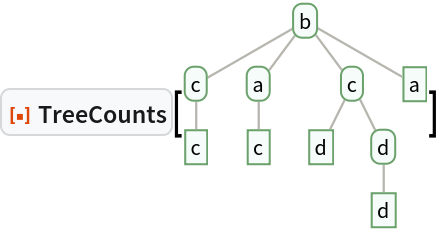Out=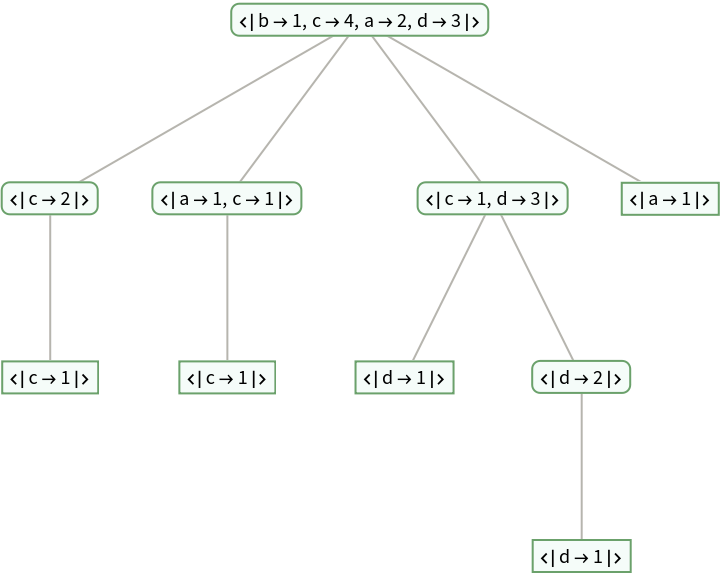### Properties and Relations (3)

Counts gives an Association of the counts of the distinct elements of a list:

 In:=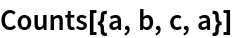Out=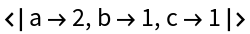TreeCounts gives an Association of the counts of the distinct data of each subtree at each node of a tree:

 In:=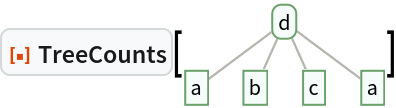Out=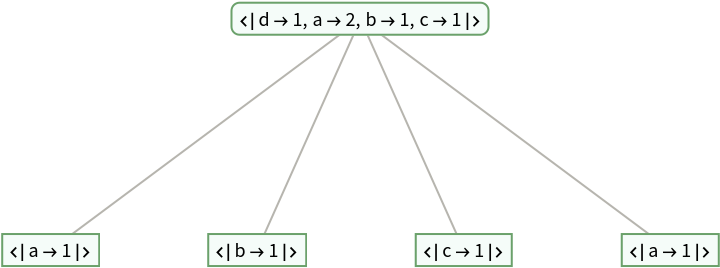Use Merge to merge two associations, using Total to combine elements with the same key:

 In:=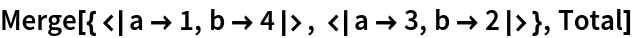Out=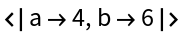TreeCounts automatically merges the counts from sibling branches:

 In:=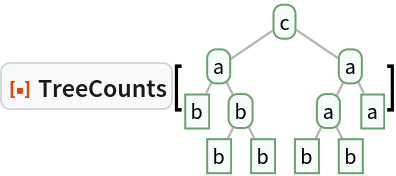Out=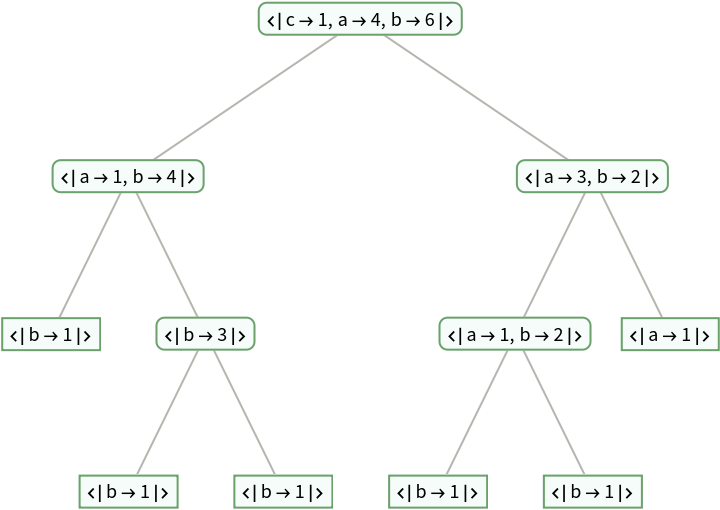TreeCount gives the total number of times a pattern matches the data in a tree:

 In:=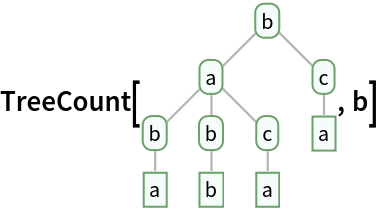Out=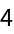TreeCounts gives the counts of the distinct data for each branch of a tree:

 In:=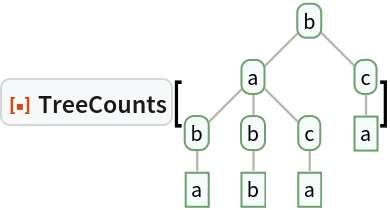Out=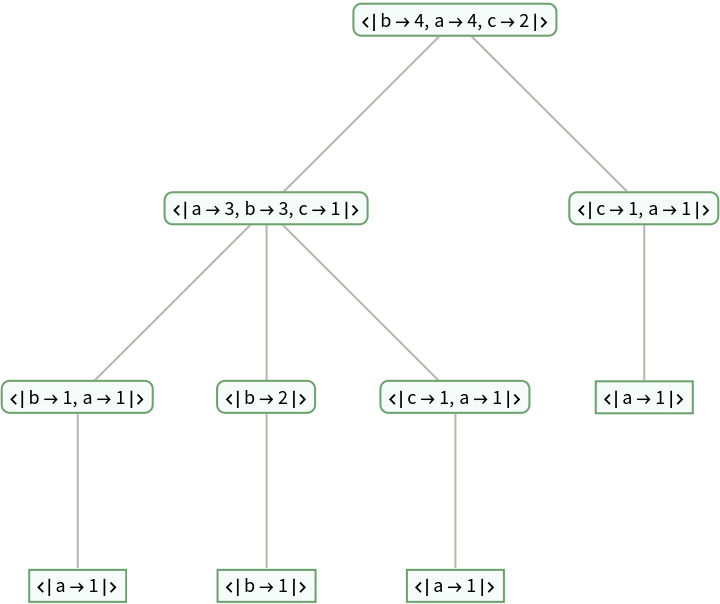## Version History

• 1.0.0 – 19 May 2023